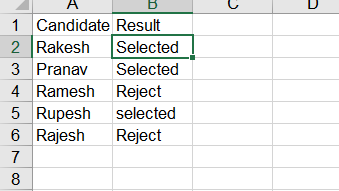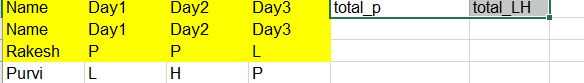# Excel logic problem

Hi All,

Below is the screenshot of excel data, i want to find the cell value whose value is Selected,.means i want cell value as 2,3, and 5 how to achieve it.

Thank you

@Rakesh_Tiwari

Use Filter Datatable
ColumnName = “Selected”

For Index of the rows

Then use For Each row activity
Declare a variable for Index
Write a If ocndition as row(“Result”).Equals(“Selected”)
True->Print the Value Else skip

Thanks

2 Likes

1. Create a variable on variables panel

Variable name - lstIndex
Variable type - list

2. Use for each row activity and pass dt

3. Use an if activity and in the condition give currentrowrow(“Result”).tostring.trim.tolower.equals(“selected”)

4. Inside the if use add to collection activity and pass item as dt.rows.indexof(currentrow) and collection as lstIndex

At the end of the process you have a list with all the row indexes of selected result.

Thanks

Hi,

check my code, it doesn’t give me the desired o/p

i followed the direction suggested by @Srini84

@Rakesh_Tiwari

Sorry, I missed the .ToString

Write as CurrentRow(“Result”).ToString.Equals(“Selected”)

Also, you can write as

CurrentRow(“Result”).ToString.ToLower.Equals(“selected”)

Try this way and let us know

Thanks

it is giving 0 and 1 value.

And add header option is checked, it should give 2,3 and 5.

@Rakesh_Tiwari

As the index starts from 0 and you enabled the Add Header Option

So the first row will become the valid as 0

To make inline to excel then you have to add Indexvalue+2

Thanks

ok, after adding indexValue+2, it gives only 2 cell value 2 and 3 what about 5?

@Rakesh_Tiwari

Did you add the .ToLower and equals as (“selected”) in lowercase?

In the 5 you can see it is lower case so we need to give as above

If so provide me the screenshot what you have done

Thanks

ok correct.

Also can you help me with another problem?

there is two sheet which is having below datanow i want to calculate total no of P and L+H in each row.

it should write the total no of p and L+H in second sheet(like total_p= 2 total-LH=1 for first row and for second row total_p= 1 and total-LH= 2)
TotalNoOfLeaves.xaml (14.0 KB)

You can also do it using lambda reference. It works quicker
indexes has type Int32

2 Likes
1 Like

You should assing total_p and total_lh before for each!

Hi @YoungFave ,

i have less knowledge in lambda function, so i don’t understand the expression.

And as you told that i should assign(total_p and total_lh) before for each so i have assigned before for each activity .

You can do:

For each row
If (CStr(row(1)) = “P” Or CStr(row(2)) = “P” Or CStr(row(3)) = “P”) Then Increment rows with P
If (CStr(row(1)) = “L” Or CStr(row(2)) = “L” Or CStr(row(3)) = “L”) AndAlso (CStr(row(1)) = “H” Or CStr(row(2)) = “H” Or CStr(row(3)) = “H”) Then Increment rows with L and H

1 Like

Hi, Thanks for your effort i used if condition suggested by you, but no luck.

Actually when i try to update the row column there i get error that the column itself not present, don’t know why.

You can specify range manually, e.g. “A1:E10”